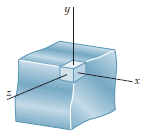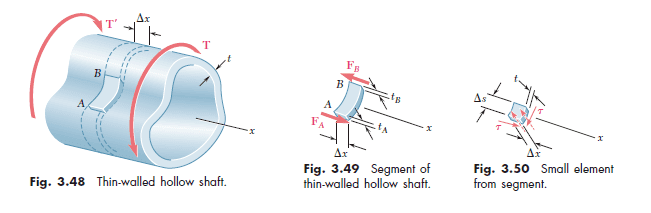# Shear stress on surface of a bar

• harmyder

## Homework Statement

I want to understand why in noncicular members in torsion book tells me that surface of a member is free and so no shearing stresses there.

This is from wikipedia for shafts:
Note that the highest shear stress occurs on the surface of the shaft, where the radius is maximum.

Why then for noncircular members it isn't so?

## Homework EquationsFrom the book:
Consider a small cubic element located at a corner of the cross section of a square bar in torsion and select coordinate axes parallel to the edges of the element. Since the face of the element perpendicular to the y-axis is part of the free surface of the bar, all stresses on this face must be zero.

## The Attempt at a Solution

On wikipedia I've read that free surface is a notion relative to fluids not solid members. And i can't understand why we have free surface on the edges of a bar not at other parts of its surface.

Last edited:
I'm currently reading Beer MoM book. There is nothing new for me in this tutorial.

I'm particulary like to understand this passage:
Since the face of the element perpendicular to the y-axis is part of the free surface of the bar, all stresses on this face must be zero.

## Homework Statement

I want to understand why in noncicular members in torsion book tells me that surface of a member is free and so no shearing stresses there.
If your book says that, it's wrong. Even for non-circular sections, the maximum shearing stress will occur somewhere on the outer surface of the shaft.

It could be that you are confusing the boundary condition of the general torsion problem with the calculation of shear stress at a particular point on the surface of the section.

The attached article discusses the shear stress of rectangular sections under torsion, and it specifically states on the bottom of p. 58 in Fig. 6 that the maximum shear stress occurs on the surface in the middle of the longer sides of the cross section (See Fig. 6(b)).

http://www.engineeringmechanics.cz/pdf/19_1_045.pdf

•harmyder

Yeh, the books tells the same about the position of the maximum shear stress. It says about free surface only for the edges.

Yeh, the books tells the same about the position of the maximum shear stress. It says about free surface only for the edges.
On the surface of the bar, there can be no tractive stresses (which is different from saying there is no shear stress).

That's what the boundary conditions listed in (6) on p. 47 mean

•harmyder
Now I'm reading part THIN-WALLED HOLLOW SHAFTSThe book says this
We now detach a small element from the wall portion AB (Fig. 201 3.50). Since the upper and lower faces of this element are part of the free surface of the hollow member, the stresses on these faces are equal to zero.

Why its surface is free?

For the case with a bar the book from the fact that surface is free concludes that shear stress are all zero on the edges.

Now I'm reading part THIN-WALLED HOLLOW SHAFTS

View attachment 101424

The book says this
We now detach a small element from the wall portion AB (Fig. 201 3.50). Since the upper and lower faces of this element are part of the free surface of the hollow member, the stresses on these faces are equal to zero.

Why its surface is free?

For the case with a bar the book from the fact that surface is free concludes that shear stress are all zero on the edges.
The surface of the shaft at the portion AB and the corresponding surface located below AB by the thickness of the outer wall are free surfaces for the simple reason they are not restrained in any way.

However, when the book says that the stresses on these faces are equal to zero, it fails to emphasize that the axial and bending stresses are zero, since the shaft is in pure torsion. More generally, the net axial force acting on the shaft is zero.

Equilibrium considerations require that FA + FB = 0, and the same will apply to the shearing forces acting along arc AB and the corresponding arc located Δx away. Those shearing forces have magnitude τ ⋅ t ⋅ Δs, using the diagram in Fig. 3.50. For thin-walled structures, it is assumed that the shearing stress τ is constant across the thickness t of the wall.

The shearing stresses in the wall are acting in a plane which is perpendicular to the axis of twist, so there will be no axial force component created by them, and the axial stresses acting on the free surfaces of the shaft remain equal to zero.

•harmyder
Which shear component of the stress tensor are you talking about? Certainly on an element of area on the surface of the bar, the air exerts no component of stress tangent to the surface.

Which shear component of the stress tensor are you talking about? Certainly on an element of area on the surface of the bar, the air exerts no component of stress tangent to the surface.

Thank you for the reply. But we have shear stress in the middle of bar side. The problem for me is that regarding edges the author says that surface is free and he concludes from it that shear stresses are zeros there. But why then surface is not free in the middle of bar side? Certainly, the air 'exerts' the same tangent stress there.

Revelation: Woow, can it be because surface in the middle of bar is curved and near the edges is not?

Last edited:
Shear stress is not something which is acting between the bar surface and the air - it is something acting within the material of the bar .

Consider a little cube of material in the outer surface of the bar .

When a torsion load is applied the bar twists and the cube becomes distorted .

(1) What stresses must then be acting on the faces of the cube ?

(2) How do these stresses vary depending on where the cube is located ?